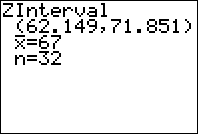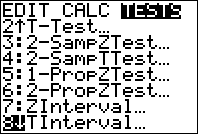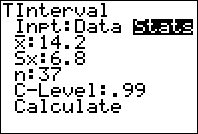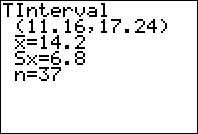﻿ Confidence Intervals for the Mean Using the TI83 or 84 Graphing Calculator - MathBootCamps

# Confidence Intervals for the Mean Using the TI83 or 84 Graphing Calculator

In order to estimate the value of an unknown population mean, we can use a confidence interval based on the standard normal distribution (z-interval) or the t-distribution (t-interval). The choice is usually determined by the following rule:

• Use a z-interval if: the population standard deviation is known and either the population is normally distributed or the sample size is larger than 30.
• Use a t-interval if: the population standard deviation is unknown and either the population is normally distributed or the sample size is larger than 30.

In both cases, you can either use the formula to compute the interval by hand or use a graphing calculator (or other software). In this article, we will see how to use the TI83/84 calculator to calculate z and t intervals. Note: You can scroll down to see a video of these steps!

## z-Intervals

A psychologist wants to estimate the amount of time college students spend browsing the internet each day. In a sample of 32 college students, the average time spent browsing the internet each day was about 67 minutes. If it is believed that the population standard deviation is 14 minutes, then calculate a 95% confidence interval to estimate the average time spent by college students browsing the internet each day.

In this example, we are told that the population standard deviation is thought to be 14 minutes and we have a large enough sample size. Therefore, a z-interval can be used to calculate the confidence interval.

### Step 1: Go to the z-interval on the calculator.

Press [STAT]->Calc->7. Z-interval [ENTER]### Step 2: Highlight STATS

Since we have statistics for the sample already calculated, we will highlight STATS at the top. If you had a list of data instead, you could enter it into L1 and then use DATA. This is really only useful for small data sets.### Step 3: Enter Data

Notice that the calculator asks for sigma (the population standard deviation) – this should help you remember that you shouldn’t be using this unless you have sigma already.### Step 4: Calculate and interpret

Please make sure to read the explanation of how to interpret intervals in general. It is very easy to misunderstand what they truly mean! Highlight CALCULATE and then press ENTER to get your interval.Here we are given our 95% confidence interval as (62.149, 71.851). This means that we are 95% confident that the true mean time that college students spend browsing the internet each day is between 62.15 minutes and 71.85 minutes. Note – there are many other ways to write this interval.

## t-intervals

When the population standard deviation is unknown, we will use a t-interval to estimate the mean. Using a t-interval on the calculator has almost the same steps as using a z-interval, as you will see below.

For a statistics project, a student samples 37 high school teachers in her community and records how many years they have worked in education. In her sample, the average number of years was 14.2 with a standard deviation of 6.8. Use this information to estimate the mean number of years worked in education for all high school teachers in the community by calculating a 99% confidence interval.

Notice that in this example, the standard deviation is coming from the sample. Therefore, this is not sigma (the population standard deviation) but instead s (the sample standard deviation). This means we should use a t-interval for this estimate.

### Step 1: Go to the t-interval on the calculator.

Press [STAT]->Calc->8. t-interval then [ENTER].### Step 2: Highlight STATS

Since we have statistics for the sample already calculated, we will highlight STATS at the top. If you had a list of data instead, you could enter it into L1 and then use DATA. This is really only useful for small data sets.### Step 3: Enter Data

Notice this time that the calculator asks for s – the sample standard deviation.### Step 4: Calculate and interpret

All you have to do is highlight CALCULATE and press ENTER.So our 99% confidence interval is (11.16, 17.24). We can interpret this by saying “We are 99% confident that the mean number of years spent working in education by high school teachers in this community is between 11.16 years and 17.24 years.”

## Video walkthrough

The following video will walk you through the steps for a t-interval or z-interval on the TI83/84 calculator.## Subscribe to our Newsletter!

We are always posting new free lessons and adding more study guides, calculator guides, and problem packs.

Sign up to get occasional emails (once every couple or three weeks) letting you know what's new!